Symmetry & Combinatorics

# Brief Notes on Symmetry & Combinatorics

#### Top Of Page

TABLE OF CONTENT
Topic #1Tri-Octo Symmetry
Topic #3{ 24-Structural Symmetry }
Topic #4Multiplex Logical Symmetry
Topic #5Concluding Remarks

### Topic #1 --- Tri-Octo Symmetry :

Let us first bring-in a 3-D { Cube } , as per the diagram on-the-left , below .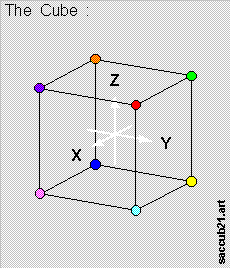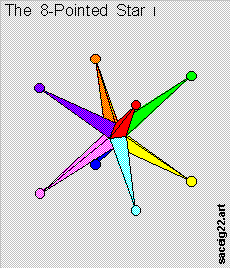Let us now construct a { 8-Pointed Star } , using the 8 corners of the cube as the { points } , as per the diagram on-the-right , above .

• The { 8-Pointed Star } is [ Octo-Symmetric ] , obviously & [ a priori ] .

Let us now rotate the { 8-Pointed Star } about the { [ -1, -1, -1 ] - [ +1, +1, +1 ] } axis ,

• thru the angles of [ 120 degrees ] , [ 240 degrees ] , & [ 360 degrees ] ;

as per the set of 4 diagrams below .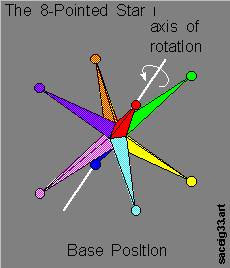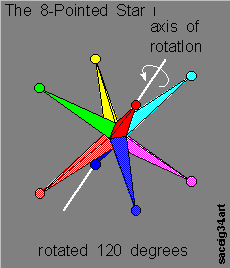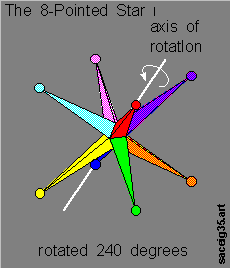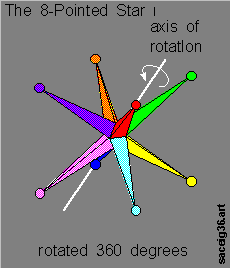• And the shape of the object remains constant throughout .

The { 8-Pointed Star } is therefore also [ Tri-Symmetric ] about 4 axis :

• the { [ -1, -1 , -1 ] - [ +1, +1, +1 ] } axis ,
• the { [ -1, -1 , +1 ] - [ +1, +1, -1 ] } axis ,
• the { [ -1, +1 , -1 ] - [ +1, -1, +1 ] } axis , &
• the { [ +1, -1 , -1 ] - [ -1, +1, +1 ] } axis .

i.e. : the 4 cross-diagonals of the { Cube } .

### Topic #2 --- Quad-Hexa Symmetry :

Let us bring- in again a 3-D { Cube } , as per the diagram on-the-left , below .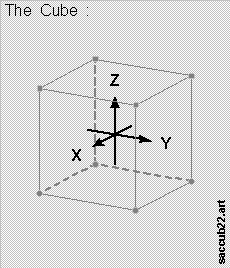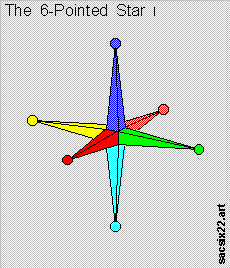Let us now construct a { 6-Pointed Star } , using the 3 orthogonal [ axis-directions ] , as per the diagram on-the-right , above .

• The { 6-Pointed Star } is [ Hexa-Symmetric ] , obviously & [ a priori ] .

Let us now rotate the { 6-Pointed Star } about [ X-Axis ] ,

• thru the angles of [ 90 degrees ] , [ 180 degrees ] , [ 270 degrees ] , & [ 360 degrees ] ;

as per the set of 4 diagrams below .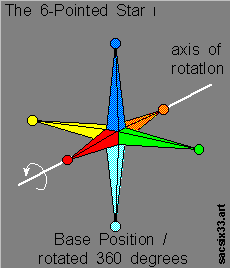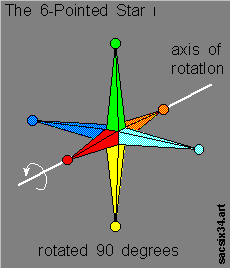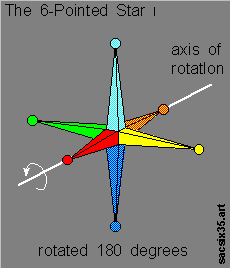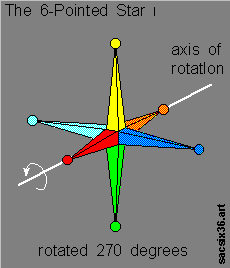• And the shape of the object remains constant throughout .

The { 6-Pointed Star } is therefore also [ Quad-Symmetric ] about 3 axial-directions , i.e. :

• the [ X-Axis ] , the [ Y-Axis ] , & the [ Z-Axis ] .

### Topic #3 --- { 24-Structural Symmetry } :

Let us now bring-in a { X-Y-Z Reference Frame } , as per the diagram on-the-left , below :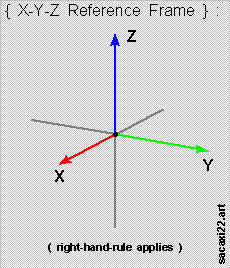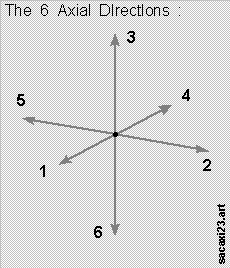Let as also identify six (6) fixed directions , as per diagram on-the-right , above , for mapping purposes .

There are then 24 ways that we can orient the { X-Y-Z Reference Frame } , mapping onto these 6 fixed-directions ,

• as per this diagram below :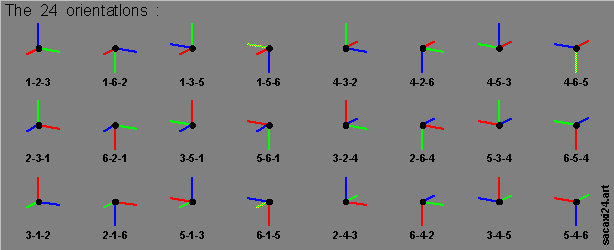Let us suppose that :

• we were able construct an object using an initial { X-Y-Z Reference Frame } as the orientation reference ;

• and subsequently , we were able to re-orient the { X-Y-Z Reference Frame } with the object attached , onto any one of the other 23 orientations .

IF we found that the shape of the object remains constant throughout ,

• regardless of which of the 24 orientions the { X-Y-Z Reference Frame } is oriented at ,

THEN the object is said-to-be [ 24-Structural Symmetric ] .

And we offer three (3) such objects with this special property :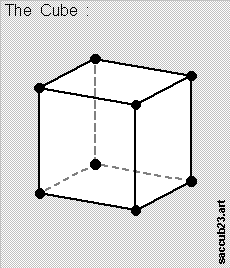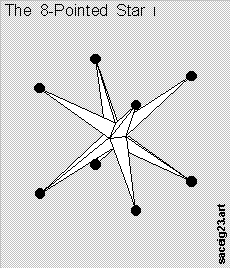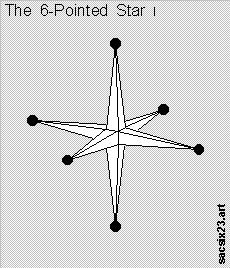• namely , the { Cube } , the { 8-Pointed Star } , & the { 6-Pointed Star } .

### Topic #4 --- Multiplex Logical Symmetry :

Let us now identify a set of eight (8) separate colors for our use in this section , namely :

• red / light-blue / purple / pink / green / yellow / orange / navy-blue .

Let us now bring-in a blank { 8-Pointed Star } to be colored , as per the diagram on-the-left , below .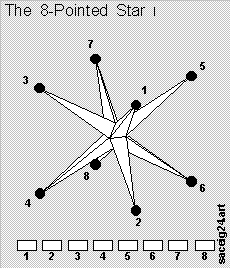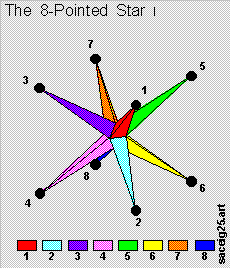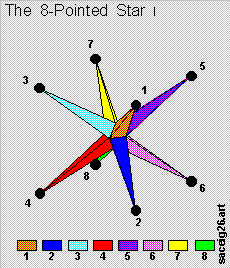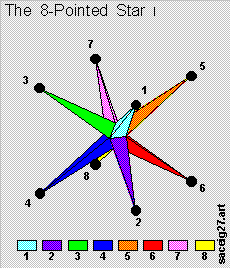Let us now paint each of the 8 arms of the { 8-Pointed Star } a different color , as per the 3 examples in the 3 diagrams on-the-right , above .

We simply note here that there are 40,320 ways , or [ 8-factorial ] ways , to do this ,

• and these can be broken-down into 1,680 sets of [ 24 members ] each ;

• with each individual set of [ 24 members ] being { 24-Structural Symmetric } within the set .

And these 40,320 configurations are said-to-be [ Logically Symmetric ] , because :

• we can always move from [ one configuration ] to [ another configuration ] simply by changing the coloring-scheme ,

• but without any changes to the orientation of the object , i.e. without implementing any [ structural changes ] .

Let us now add a little twist & spice , by coloring the 8 { nodal points } the same color as the arm ,

• except for an opposite pair of arms , namely the { red-color-arm } & the { blue-color-arm } , where we swapped colors for the { nodal points } ,

as per the diagram of-the-left , below , i.e. { Diagram LS-1 } .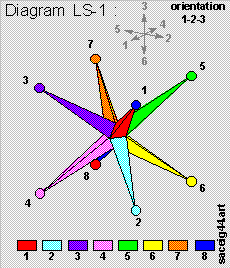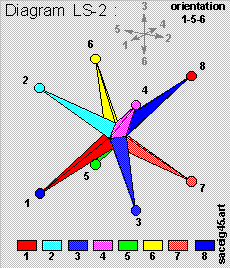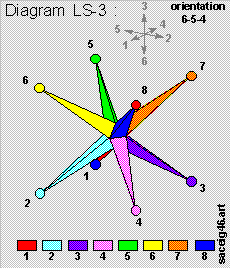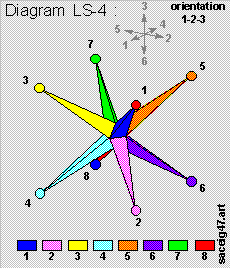Let us now rotate the entire [ object ] about the { "1"-axis } , as marked { grey-color } ,

• counter-clockwise 180 degrees , to arrive at the configuration in { Diagram LS-2 } above .

Let us again rotate the entire [ object ] about the { "2"-axis } , as marked { grey-color } ,

• counter-clockwise 90 degrees , to arrive at the configuration in { Diagram LS-3 } above .

Let us now try to arrive the configuration in { Diagram LS-3 } via the [ logical ] route , i.e. :

• starting with the coloring-scheme as per the bottom-row in { Diagram LS-1 } , and

changing the coloring-scheme to the one as per the bottom-row in { Diagram LS-4 } , above .

And the configurations in { Diagram LS-3 } & { Diagram LS-4 } are now the same . Interesting eh !

Let us try another configuration , as per the { Diagram LS-5 } on-the-left , below .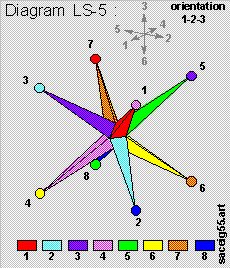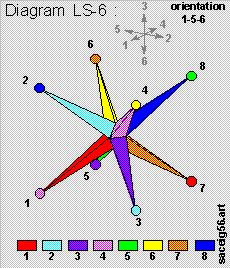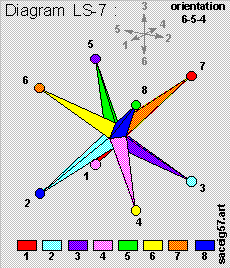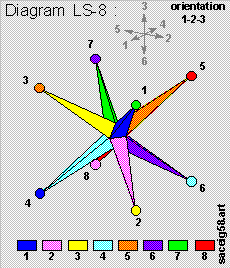Let us now rotate the entire [ object ] about the { "1"-axis } , as marked { grey-color } ,

• counter-clockwise 180 degrees , to arrive at the configuration in { Diagram LS-6 } above .

Let us again rotate the entire [ object ] about the { "2"-axis } , as marked { grey-color } ,

• counter-clockwise 90 degrees , to arrive at the configuration in { Diagram LS-7 } above .

Let us now try to arrive the configuration in { Diagram LS-7 } via the [ logical ] route , i.e. :

• starting with the coloring-scheme as per the bottom-row in { Diagram LS-5 } , and

changing the coloring-scheme to the one as per the bottom-row in { Diagram LS-8 } , above .

And the configurations in { Diagram LS-7 } & { Diagram LS-8 } are now the same .

( Hint : try the [ 1-4-6-7 tetrahedron ] symmetry vs. the [ 8-5-3-2 tetrahedron ] symmetry . )

We also note here , in passing , that :

• for the base configuration in { Diagram LS-1 } , of the other 23 configurations arising from [ 24-Structural Symmerty ] ,

• only 5 of the 23 configurations exhibit [ logical symmetry ] with respect to the [ base configuration ] ;

• for the base configuration in { Diagram LS-5 } , of the other 23 configurations arising from [ 24-Structural Symmerty ] ,

• the entire 23 configurations exhibit [ logical symmetry ] with respect to the [ base configuration ] .

Symmetry , sometimes , can be more deceptive than first-meet-the-eye .

### Topic #5 --- Concluding Remarks :

The arithmetic equation for { Tri-Octo Symmetry } & { Quad-Hexa Symmetry } is quite naturally :

• 24 = 3 x 8 = 4 x 6

And we now make this observation :

• The "I-CHING" started-off with 8 { Trigrams } of 3-lines each ,

and then moves-on to the 64 { Hexagrams } of 6-lines each ,

• via a [ quad / modulo 4 ] procedure , i.e. the { Ceremony with the 50-sticks } .

The "I-CHING" may , therefore , have been rooted in the study of symmetry & assymetry of geometric shapes ,

• occuring naturally and otherwise .

And more effort in this direction may be warranted .

Finally , we bring-in the { Equilateral Tetrahedron } & the { 4-Pointed Star } :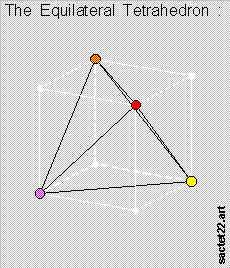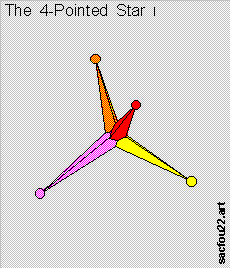• for the reader's further thoughts .